Chemistry 2e

# Exercises

Chemistry 2eExercises

### 12.1Chemical Reaction Rates

1.

What is the difference between average rate, initial rate, and instantaneous rate?

2.

Ozone decomposes to oxygen according to the equation $2O3(g)⟶3O2(g).2O3(g)⟶3O2(g).$ Write the equation that relates the rate expressions for this reaction in terms of the disappearance of O3 and the formation of oxygen.

3.

In the nuclear industry, chlorine trifluoride is used to prepare uranium hexafluoride, a volatile compound of uranium used in the separation of uranium isotopes. Chlorine trifluoride is prepared by the reaction $Cl2(g)+3F2(g)⟶2ClF3(g).Cl2(g)+3F2(g)⟶2ClF3(g).$ Write the equation that relates the rate expressions for this reaction in terms of the disappearance of Cl2 and F2 and the formation of ClF3.

4.

A study of the rate of dimerization of C4H6 gave the data shown in the table:
$2C4H6⟶C8H122C4H6⟶C8H12$

 Time (s) 0 1600 3200 4800 6200 [C4H6] (M) 1.00 $××$ 10−2 5.04 $××$ 10−3 3.37 $××$ 10−3 2.53 $××$ 10−3 2.08 $××$ 10−3

(a) Determine the average rate of dimerization between 0 s and 1600 s, and between 1600 s and 3200 s.

(b) Estimate the instantaneous rate of dimerization at 3200 s from a graph of time versus [C4H6]. What are the units of this rate?

(c) Determine the average rate of formation of C8H12 at 1600 s and the instantaneous rate of formation at 3200 s from the rates found in parts (a) and (b).

5.

A study of the rate of the reaction represented as $2A⟶B2A⟶B$ gave the following data:

 Time (s) 0 5 10 15 20 25 35 [A] (M) 1 0.775 0.625 0.465 0.36 0.285 0.23

(a) Determine the average rate of disappearance of A between 0.0 s and 10.0 s, and between 10.0 s and 20.0 s.

(b) Estimate the instantaneous rate of disappearance of A at 15.0 s from a graph of time versus [A]. What are the units of this rate?

(c) Use the rates found in parts (a) and (b) to determine the average rate of formation of B between 0.00 s and 10.0 s, and the instantaneous rate of formation of B at 15.0 s.

6.

Consider the following reaction in aqueous solution:
$5Br−(aq)+BrO3−(aq)+6H+(aq)⟶3Br2(aq)+3H2O(l)5Br−(aq)+BrO3−(aq)+6H+(aq)⟶3Br2(aq)+3H2O(l)$

If the rate of disappearance of Br(aq) at a particular moment during the reaction is 3.5 $××$ 10−4 mol L−1 s−1, what is the rate of appearance of Br2(aq) at that moment?

### 12.2Factors Affecting Reaction Rates

7.

Describe the effect of each of the following on the rate of the reaction of magnesium metal with a solution of hydrochloric acid: the molarity of the hydrochloric acid, the temperature of the solution, and the size of the pieces of magnesium.

8.

Explain why an egg cooks more slowly in boiling water in Denver than in New York City. (Hint: Consider the effect of temperature on reaction rate and the effect of pressure on boiling point.)

9.

Go to the PhET Reactions & Rates interactive. Use the Single Collision tab to represent how the collision between monatomic oxygen (O) and carbon monoxide (CO) results in the breaking of one bond and the formation of another. Pull back on the red plunger to release the atom and observe the results. Then, click on “Reload Launcher” and change to “Angled shot” to see the difference.

(a) What happens when the angle of the collision is changed?

(b) Explain how this is relevant to rate of reaction.

10.

In the PhET Reactions & Rates interactive, use the “Many Collisions” tab to observe how multiple atoms and molecules interact under varying conditions. Select a molecule to pump into the chamber. Set the initial temperature and select the current amounts of each reactant. Select “Show bonds” under Options. How is the rate of the reaction affected by concentration and temperature?

11.

In the PhET Reactions & Rates interactive, on the Many Collisions tab, set up a simulation with 15 molecules of A and 10 molecules of BC. Select “Show Bonds” under Options.

(a) Leave the Initial Temperature at the default setting. Observe the reaction. Is the rate of reaction fast or slow?

(b) Click “Pause” and then “Reset All,” and then enter 15 molecules of A and 10 molecules of BC once again. Select “Show Bonds” under Options. This time, increase the initial temperature until, on the graph, the total average energy line is completely above the potential energy curve. Describe what happens to the reaction.

### 12.3Rate Laws

12.

How do the rate of a reaction and its rate constant differ?

13.

Doubling the concentration of a reactant increases the rate of a reaction four times. With this knowledge, answer the following questions:

(a) What is the order of the reaction with respect to that reactant?

(b) Tripling the concentration of a different reactant increases the rate of a reaction three times. What is the order of the reaction with respect to that reactant?

14.

Tripling the concentration of a reactant increases the rate of a reaction nine-fold. With this knowledge, answer the following questions:

(a) What is the order of the reaction with respect to that reactant?

(b) Increasing the concentration of a reactant by a factor of four increases the rate of a reaction four-fold. What is the order of the reaction with respect to that reactant?

15.

How will the rate of reaction change for the process: $CO(g)+NO2(g)⟶CO2(g)+NO(g)CO(g)+NO2(g)⟶CO2(g)+NO(g)$ if the rate law for the reaction is $rate=k[NO2]2?rate=k[NO2]2?$

(a) Decreasing the pressure of NO2 from 0.50 atm to 0.250 atm.

(b) Increasing the concentration of CO from 0.01 M to 0.03 M.

16.

How will each of the following affect the rate of the reaction: $CO(g)+NO2(g)⟶CO2(g)+NO(g)CO(g)+NO2(g)⟶CO2(g)+NO(g)$ if the rate law for the reaction is $rate=k[NO2][CO]rate=k[NO2][CO]$?

(a) Increasing the pressure of NO2 from 0.1 atm to 0.3 atm

(b) Increasing the concentration of CO from 0.02 M to 0.06 M.

17.

Regular flights of supersonic aircraft in the stratosphere are of concern because such aircraft produce nitric oxide, NO, as a byproduct in the exhaust of their engines. Nitric oxide reacts with ozone, and it has been suggested that this could contribute to depletion of the ozone layer. The reaction $NO+O3⟶NO2+O2NO+O3⟶NO2+O2$ is first order with respect to both NO and O3 with a rate constant of 2.20 $××$ 107 L/mol/s. What is the instantaneous rate of disappearance of NO when [NO] = 3.3 $××$ 10−6 M and [O3] = 5.9 $××$ 10−7 M?

18.

Radioactive phosphorus is used in the study of biochemical reaction mechanisms because phosphorus atoms are components of many biochemical molecules. The location of the phosphorus (and the location of the molecule it is bound in) can be detected from the electrons (beta particles) it produces:
$1532P⟶ 1632S+e−1532P⟶ 1632S+e−$
rate = 4.85 $××$ 10−2 $day−1[32P]day−1[32P]$

What is the instantaneous rate of production of electrons in a sample with a phosphorus concentration of 0.0033 M?

19.

The rate constant for the radioactive decay of 14C is 1.21 $××$ 10−4 year−1. The products of the decay are nitrogen atoms and electrons (beta particles):
$614C⟶714N+e−614C⟶714N+e−$
$rate=k[614C]rate=k[614C]$

What is the instantaneous rate of production of N atoms in a sample with a carbon-14 content of 6.5 $××$ 10−9 M?

20.

The decomposition of acetaldehyde is a second order reaction with a rate constant of 4.71 $××$ 10−8 L mol−1 s−1. What is the instantaneous rate of decomposition of acetaldehyde in a solution with a concentration of 5.55 $××$ 10−4 M?

21.

Alcohol is removed from the bloodstream by a series of metabolic reactions. The first reaction produces acetaldehyde; then other products are formed. The following data have been determined for the rate at which alcohol is removed from the blood of an average male, although individual rates can vary by 25–30%. Women metabolize alcohol a little more slowly than men:

 [C2H5OH] (M) 4.4 $××$ 10−2 3.3 $××$ 10−2 2.2 $××$ 10−2 Rate (mol L−1 h−1) 2.0 $××$ 10−2 2.0 $××$ 10−2 2.0 $××$ 10−2

Determine the rate law, the rate constant, and the overall order for this reaction.

22.

Under certain conditions the decomposition of ammonia on a metal surface gives the following data:

 [NH3] (M) 1.0 $××$ 10−3 2.0 $××$ 10−3 3.0 $××$ 10−3 Rate (mol L−1 h−1) 1.5 $××$ 10−6 1.5 $××$ 10−6 1.5 $××$ 10−6

Determine the rate law, the rate constant, and the overall order for this reaction.

23.

Nitrosyl chloride, NOCl, decomposes to NO and Cl2.
$2NOCl(g)⟶2NO(g)+Cl2(g)2NOCl(g)⟶2NO(g)+Cl2(g)$

Determine the rate law, the rate constant, and the overall order for this reaction from the following data:

 [NOCl] (M) 0.10 0.20 0.30 Rate (mol L−1 h−1) 8.0 $××$ 10−10 3.2 $××$ 10−9 7.2 $××$ 10−9
24.

From the following data, determine the rate law, the rate constant, and the order with respect to A for the reaction $A⟶2C.A⟶2C.$

 [A] (M) 1.33 $××$ 10−2 2.66 $××$ 10−2 3.99 $××$ 10−2 Rate (mol L−1 h−1) 3.80 $××$ 10−7 1.52 $××$ 10−6 3.42 $××$ 10−6
25.

Nitrogen monoxide reacts with chlorine according to the equation:
$2NO(g)+Cl2(g)⟶2NOCl(g)2NO(g)+Cl2(g)⟶2NOCl(g)$

The following initial rates of reaction have been observed for certain reactant concentrations:

[NO] (mol/L) [Cl2] (mol/L) Rate (mol L−1 h−1)
0.50 0.50 1.14
1.00 0.50 4.56
1.00 1.00 9.12

What is the rate law that describes the rate’s dependence on the concentrations of NO and Cl2? What is the rate constant? What are the orders with respect to each reactant?

26.

Hydrogen reacts with nitrogen monoxide to form dinitrogen monoxide (laughing gas) according to the equation: $H2(g)+2NO(g)⟶N2O(g)+H2O(g)H2(g)+2NO(g)⟶N2O(g)+H2O(g)$

Determine the rate law, the rate constant, and the orders with respect to each reactant from the following data:

 [NO] (M) 0.30 0.60 0.60 [H2] (M) 0.35 0.35 0.70 Rate (mol L−1 s−1) 2.835 $××$ 10−3 1.134 $××$ 10−2 2.268 $××$ 10−2
27.

For the reaction $A⟶B+C,A⟶B+C,$ the following data were obtained at 30 °C:

 [A] (M) 0.230 0.356 0.557 Rate (mol L−1 s−1) 4.17 $××$ 10−4 9.99 $××$ 10−4 2.44 $××$ 10−3

(a) What is the order of the reaction with respect to [A], and what is the rate law?

(b) What is the rate constant?

28.

For the reaction $Q⟶W+X,Q⟶W+X,$ the following data were obtained at 30 °C:

 [Q]initial (M) 0.170 0.212 0.357 Rate (mol L−1 s−1) 6.68 $××$ 10−3 1.04 $××$ 10−2 2.94 $××$ 10−2

(a) What is the order of the reaction with respect to [Q], and what is the rate law?

(b) What is the rate constant?

29.

The rate constant for the first-order decomposition at 45 °C of dinitrogen pentoxide, N2O5, dissolved in chloroform, CHCl3, is 6.2 $××$ 10−4 min−1.
$2N2O5⟶4NO2+O22N2O5⟶4NO2+O2$

What is the rate of the reaction when [N2O5] = 0.40 M?

30.

The annual production of HNO3 in 2013 was 60 million metric tons Most of that was prepared by the following sequence of reactions, each run in a separate reaction vessel.

(a) $4NH3(g)+5O2(g)⟶4NO(g)+6H2O(g)4NH3(g)+5O2(g)⟶4NO(g)+6H2O(g)$

(b) $2NO(g)+O2(g)⟶2NO2(g)2NO(g)+O2(g)⟶2NO2(g)$

(c) $3NO2(g)+H2O(l)⟶2HNO3(aq)+NO(g)3NO2(g)+H2O(l)⟶2HNO3(aq)+NO(g)$

The first reaction is run by burning ammonia in air over a platinum catalyst. This reaction is fast. The reaction in equation (c) is also fast. The second reaction limits the rate at which nitric acid can be prepared from ammonia. If equation (b) is second order in NO and first order in O2, what is the rate of formation of NO2 when the oxygen concentration is 0.50 M and the nitric oxide concentration is 0.75 M? The rate constant for the reaction is 5.8 $××$ 10−6 L2 mol−2 s−1.

31.

The following data have been determined for the reaction:
$I−+OCl−⟶IO−+Cl−I−+OCl−⟶IO−+Cl−$

1 2 3
$[I−]initial[I−]initial$ (M) 0.10 0.20 0.30
$[OCl−]initial[OCl−]initial$ (M) 0.050 0.050 0.010
Rate (mol L−1 s−1) 3.05 $××$ 10−4 6.20 $××$ 10−4 1.83 $××$ 10−4

Determine the rate law and the rate constant for this reaction.

### 12.4Integrated Rate Laws

32.

Describe how graphical methods can be used to determine the order of a reaction and its rate constant from a series of data that includes the concentration of A at varying times.

33.

Use the data provided to graphically determine the order and rate constant of the following reaction: $SO2Cl2⟶SO2+Cl2SO2Cl2⟶SO2+Cl2$

 Time (s) 0 5.00 $××$ 103 1.00 $××$ 104 1.50 $××$ 104 [SO2Cl2] (M) 0.100 0.0896 0.0802 0.0719 Time (s) 2.50 $××$ 104 3.00 $××$ 104 4.00 $××$ 104 [SO2Cl2] (M) 0.0577 0.0517 0.0415
34.

Pure ozone decomposes slowly to oxygen, $2O3(g)⟶3O2(g).2O3(g)⟶3O2(g).$ Use the data provided in a graphical method and determine the order and rate constant of the reaction.

 Time (h) 0 2.0 $××$ 103 7.6 $××$ 103 1.00 $××$ 104 [O3] (M) 1.00 $××$ 10−5 4.98 $××$ 10−6 2.07 $××$ 10−6 1.66 $××$ 10−6 Time (h) 1.23 $××$ 104 1.43 $××$ 104 1.70 $××$ 104 [O3] (M) 1.39 $××$ 10−6 1.22 $××$ 10−6 1.05 $××$ 10−6
35.

From the given data, use a graphical method to determine the order and rate constant of the following reaction:
$2X⟶Y+Z2X⟶Y+Z$

 Time (s) 5 10 15 20 25 30 35 40 [X] (M) 0.099 0.0497 0.0332 0.0249 0.02 0.0166 0.0143 0.0125
36.

What is the half-life for the first-order decay of phosphorus-32? $( 1532P ⟶ 1632S +e−)(1532P⟶1632S+e−)$ The rate constant for the decay is 4.85 $××$ 10−2 day−1.

37.

What is the half-life for the first-order decay of carbon-14? $( 614C ⟶ 714N +e−)(614C⟶714N+e−)$ The rate constant for the decay is 1.21 $××$ 10−4 year−1.

38.

What is the half-life for the decomposition of NOCl when the concentration of NOCl is 0.15 M? The rate constant for this second-order reaction is 8.0 $××$ 10−8 L mol−1 s−1.

39.

What is the half-life for the decomposition of O3 when the concentration of O3 is 2.35 $××$ 10−6 M? The rate constant for this second-order reaction is 50.4 L mol−1 h−1.

40.

The reaction of compound  A to give compounds  C and  D was found to be second-order in  A. The rate constant for the reaction was determined to be 2.42 L mol−1 s−1. If the initial concentration is 0.500 mol/L, what is the value of t1/2?

41.

The half-life of a reaction of compound A to give compounds D and E is 8.50 min when the initial concentration of A is 0.150 M. How long will it take for the concentration to drop to 0.0300 M if the reaction is (a) first order with respect to A or (b) second order with respect to A?

42.

Some bacteria are resistant to the antibiotic penicillin because they produce penicillinase, an enzyme with a molecular weight of 3 $××$ 104 g/mol that converts penicillin into inactive molecules. Although the kinetics of enzyme-catalyzed reactions can be complex, at low concentrations this reaction can be described by a rate law that is first order in the catalyst (penicillinase) and that also involves the concentration of penicillin. From the following data: 1.0 L of a solution containing 0.15 µg (0.15 $××$ 10−6 g) of penicillinase, determine the order of the reaction with respect to penicillin and the value of the rate constant.

[Penicillin] (M) Rate (mol L−1 min−1)
2.0 $××$ 10−6 1.0 $××$ 10−10
3.0 $××$ 10−6 1.5 $××$ 10−10
4.0 $××$ 10−6 2.0 $××$ 10−10
43.

Both technetium-99 and thallium-201 are used to image heart muscle in patients with suspected heart problems. The half-lives are 6 h and 73 h, respectively. What percent of the radioactivity would remain for each of the isotopes after 2 days (48 h)?

44.

There are two molecules with the formula C3H6. Propene, $CH3CH=CH2,CH3CH=CH2,$ is the monomer of the polymer polypropylene, which is used for indoor-outdoor carpets. Cyclopropane is used as an anesthetic: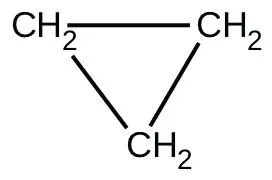When heated to 499 °C, cyclopropane rearranges (isomerizes) and forms propene with a rate constant of
5.95 $××$ 10−4 s−1. What is the half-life of this reaction? What fraction of the cyclopropane remains after 0.75 h at 499 °C?

45.

Fluorine-18 is a radioactive isotope that decays by positron emission to form oxygen-18 with a half-life of 109.7 min. (A positron is a particle with the mass of an electron and a single unit of positive charge; the equation is $918F ⟶ 818O ++10e)918F⟶818O++10e)$ Physicians use 18F to study the brain by injecting a quantity of fluoro-substituted glucose into the blood of a patient. The glucose accumulates in the regions where the brain is active and needs nourishment.

(a) What is the rate constant for the decomposition of fluorine-18?

(b) If a sample of glucose containing radioactive fluorine-18 is injected into the blood, what percent of the radioactivity will remain after 5.59 h?

(c) How long does it take for 99.99% of the 18F to decay?

46.

Suppose that the half-life of steroids taken by an athlete is 42 days. Assuming that the steroids biodegrade by a first-order process, how long would it take for $164164$ of the initial dose to remain in the athlete’s body?

47.

Recently, the skeleton of King Richard III was found under a parking lot in England. If tissue samples from the skeleton contain about 93.79% of the carbon-14 expected in living tissue, what year did King Richard III die? The half-life for carbon-14 is 5730 years.

48.

Nitroglycerine is an extremely sensitive explosive. In a series of carefully controlled experiments, samples of the explosive were heated to 160 °C and their first-order decomposition studied. Determine the average rate constants for each experiment using the following data:

 Initial [C3H5N3O9] (M) 4.88 3.52 2.29 1.81 5.33 4.05 2.95 1.72 t (s) 300 300 300 300 180 180 180 180 % Decomposed 52 52.9 53.2 53.9 34.6 35.9 36 35.4
49.

For the past 10 years, the unsaturated hydrocarbon 1,3-butadiene $(CH2=CH–CH=CH2)(CH2=CH–CH=CH2)$ has ranked 38th among the top 50 industrial chemicals. It is used primarily for the manufacture of synthetic rubber. An isomer exists also as cyclobutene: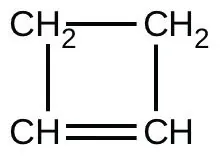The isomerization of cyclobutene to butadiene is first-order and the rate constant has been measured as 2.0 $××$ 10−4 s−1 at 150 °C in a 0.53-L flask. Determine the partial pressure of cyclobutene and its concentration after 30.0 minutes if an isomerization reaction is carried out at 150 °C with an initial pressure of 55 torr.

### 12.5Collision Theory

50.

Chemical reactions occur when reactants collide. What are two factors that may prevent a collision from producing a chemical reaction?

51.

When every collision between reactants leads to a reaction, what determines the rate at which the reaction occurs?

52.

What is the activation energy of a reaction, and how is this energy related to the activated complex of the reaction?

53.

Account for the relationship between the rate of a reaction and its activation energy.

54.

Describe how graphical methods can be used to determine the activation energy of a reaction from a series of data that includes the rate of reaction at varying temperatures.

55.

How does an increase in temperature affect rate of reaction? Explain this effect in terms of the collision theory of the reaction rate.

56.

The rate of a certain reaction doubles for every 10 °C rise in temperature.

(a) How much faster does the reaction proceed at 45 °C than at 25 °C?

(b) How much faster does the reaction proceed at 95 °C than at 25 °C?

57.

In an experiment, a sample of NaClO3 was 90% decomposed in 48 min. Approximately how long would this decomposition have taken if the sample had been heated 20 °C higher? (Hint: Assume the rate doubles for each 10 °C rise in temperature.)

58.

The rate constant at 325 °C for the decomposition reaction $C4H8⟶2C2H4C4H8⟶2C2H4$ is 6.1 $××$ 10−8 s−1, and the activation energy is 261 kJ per mole of C4H8. Determine the frequency factor for the reaction.

59.

The rate constant for the decomposition of acetaldehyde, CH3CHO, to methane, CH4, and carbon monoxide, CO, in the gas phase is 1.1 $××$ 10−2 L mol−1 s−1 at 703 K and 4.95 L mol−1 s−1 at 865 K. Determine the activation energy for this decomposition.

60.

An elevated level of the enzyme alkaline phosphatase (ALP) in human serum is an indication of possible liver or bone disorder. The level of serum ALP is so low that it is very difficult to measure directly. However, ALP catalyzes a number of reactions, and its relative concentration can be determined by measuring the rate of one of these reactions under controlled conditions. One such reaction is the conversion of p-nitrophenyl phosphate (PNPP) to p-nitrophenoxide ion (PNP) and phosphate ion. Control of temperature during the test is very important; the rate of the reaction increases 1.47 times if the temperature changes from 30 °C to 37 °C. What is the activation energy for the ALP–catalyzed conversion of PNPP to PNP and phosphate?

61.

In terms of collision theory, to which of the following is the rate of a chemical reaction proportional?

(a) the change in free energy per second

(b) the change in temperature per second

(c) the number of collisions per second

(d) the number of product molecules

62.

Hydrogen iodide, HI, decomposes in the gas phase to produce hydrogen, H2, and iodine, I2. The value of the rate constant, k, for the reaction was measured at several different temperatures and the data are shown here:

Temperature (K) k (L mol−1 s−1)
555 6.23 $××$ 10−7
575 2.42 $××$ 10−6
645 1.44 $××$ 10−4
700 2.01 $××$ 10−3

What is the value of the activation energy (in kJ/mol) for this reaction?

63.

The element Co exists in two oxidation states, Co(II) and Co(III), and the ions form many complexes. The rate at which one of the complexes of Co(III) was reduced by Fe(II) in water was measured. Determine the activation energy of the reaction from the following data:

T (K) k (s−1)
293 0.054
298 0.100
64.

The hydrolysis of the sugar sucrose to the sugars glucose and fructose,
$C12H22O11+H2O⟶C6H12O6+C6H12O6C12H22O11+H2O⟶C6H12O6+C6H12O6$

follows a first-order rate law for the disappearance of sucrose: rate = k[C12H22O11] (The products of the reaction, glucose and fructose, have the same molecular formulas but differ in the arrangement of the atoms in their molecules.)

(a) In neutral solution, k = 2.1 $××$ 10−11 s−1 at 27 °C and 8.5 $××$ 10−11 s−1 at 37 °C. Determine the activation energy, the frequency factor, and the rate constant for this equation at 47 °C (assuming the kinetics remain consistent with the Arrhenius equation at this temperature).

(b) When a solution of sucrose with an initial concentration of 0.150 M reaches equilibrium, the concentration of sucrose is 1.65 $××$ 10−7 M. How long will it take the solution to reach equilibrium at 27 °C in the absence of a catalyst? Because the concentration of sucrose at equilibrium is so low, assume that the reaction is irreversible.

(c) Why does assuming that the reaction is irreversible simplify the calculation in part (b)?

65.

Use the PhET Reactions & Rates interactive simulation to simulate a system. On the “Single collision” tab of the simulation applet, enable the “Energy view” by clicking the “+” icon. Select the first $A+BC⟶AB+CA+BC⟶AB+C$ reaction (A is yellow, B is purple, and C is navy blue). Using the “straight shot” default option, try launching the A atom with varying amounts of energy. What changes when the Total Energy line at launch is below the transition state of the Potential Energy line? Why? What happens when it is above the transition state? Why?

66.

Use the PhET Reactions & Rates interactive simulation to simulate a system. On the “Single collision” tab of the simulation applet, enable the “Energy view” by clicking the “+” icon. Select the first $A+BC⟶AB+CA+BC⟶AB+C$ reaction (A is yellow, B is purple, and C is navy blue). Using the “angled shot” option, try launching the A atom with varying angles, but with more Total energy than the transition state. What happens when the A atom hits the BC molecule from different directions? Why?

### 12.6Reaction Mechanisms

67.

Why are elementary reactions involving three or more reactants very uncommon?

68.

In general, can we predict the effect of doubling the concentration of A on the rate of the overall reaction $A+B⟶CA+B⟶C$? Can we predict the effect if the reaction is known to be an elementary reaction?

69.

Define these terms:

(a) unimolecular reaction

(b) bimolecular reaction

(c) elementary reaction

(d) overall reaction

70.

What is the rate law for the elementary termolecular reaction $A+2B⟶products?A+2B⟶products?$ For $3A⟶products?3A⟶products?$

71.

Given the following reactions and the corresponding rate laws, in which of the reactions might the elementary reaction and the overall reaction be the same?

$(a) Cl 2 + CO ⟶ Cl 2 CO rate = k [ Cl 2 ] 3/2 [ CO ] (a) Cl 2 + CO ⟶ Cl 2 CO rate = k [ Cl 2 ] 3/2 [ CO ]$

$(b) PCl 3 + Cl 2 ⟶ PCl 5 rate = k [ PCl 3 ] [ Cl 2 ] (b) PCl 3 + Cl 2 ⟶ PCl 5 rate = k [ PCl 3 ] [ Cl 2 ]$

$(c) 2 NO + H 2 ⟶ N 2 + H 2 O 2 rate = k [ NO ] [ H 2 ] (c) 2 NO + H 2 ⟶ N 2 + H 2 O 2 rate = k [ NO ] [ H 2 ]$

$(d) 2 NO + O 2 ⟶ 2 NO 2 rate = k [NO] 2 [ O 2 ] (d) 2 NO + O 2 ⟶ 2 NO 2 rate = k [NO] 2 [ O 2 ]$

$(e) NO + O 3 ⟶ NO 2 + O 2 rate = k [ NO ] [ O 3 ] (e) NO + O 3 ⟶ NO 2 + O 2 rate = k [ NO ] [ O 3 ]$

72.

Write the rate law for each of the following elementary reactions:

(a) $O3→sunlightO2+OO3→sunlightO2+O$

(b) $O3+Cl⟶O2+ClOO3+Cl⟶O2+ClO$

(c) $ClO+O⟶Cl+O2ClO+O⟶Cl+O2$

(d) $O3+NO⟶NO2+O2O3+NO⟶NO2+O2$

(e) $NO2+O⟶NO+O2NO2+O⟶NO+O2$

73.

Nitrogen monoxide, NO, reacts with hydrogen, H2, according to the following equation:
$2NO+2H2⟶N2+2H2O2NO+2H2⟶N2+2H2O$

What would the rate law be if the mechanism for this reaction were:
$2NO+H2⟶N2+H2O2(slow)H2O2+H2⟶2H2O(fast)2NO+H2⟶N2+H2O2(slow)H2O2+H2⟶2H2O(fast)$

74.

Experiments were conducted to study the rate of the reaction represented by this equation.2
$2NO(g)+2H2(g)⟶N2(g)+2H2O(g)2NO(g)+2H2(g)⟶N2(g)+2H2O(g)$

Initial concentrations and rates of reaction are given here.

Experiment Initial Concentration [NO] (mol L−1) Initial Concentration, [H2] (mol L−1 min−1) Initial Rate of Formation of N2 (mol L−1 min−1)
1 0.0060 0.0010 1.8 $××$ 10−4
2 0.0060 0.0020 3.6 $××$ 10−4
3 0.0010 0.0060 0.30 $××$ 10−4
4 0.0020 0.0060 1.2 $××$ 10−4

Consider the following questions:

(a) Determine the order for each of the reactants, NO and H2, from the data given and show your reasoning.

(b) Write the overall rate law for the reaction.

(c) Calculate the value of the rate constant, k, for the reaction. Include units.

(d) For experiment 2, calculate the concentration of NO remaining when exactly one-half of the original amount of H2 had been consumed.

(e) The following sequence of elementary steps is a proposed mechanism for the reaction.

Step 1: $NO+NO⇌N2O2NO+NO⇌N2O2$

Step 2: $N2O2+H2⇌H2O+N2ON2O2+H2⇌H2O+N2O$

Step 3: $N2O+H2⇌N2+H2ON2O+H2⇌N2+H2O$

Based on the data presented, which of these is the rate determining step? Show that the mechanism is consistent with the observed rate law for the reaction and the overall stoichiometry of the reaction.

75.

The reaction of CO with Cl2 gives phosgene (COCl2), a nerve gas that was used in World War I. Use the mechanism shown here to complete the following exercises:
$Cl2(g)⇌2Cl(g)Cl2(g)⇌2Cl(g)$ (fast, k1 represents the forward rate constant, k−1 the reverse rate constant)
$CO(g)+Cl(g)⟶COCl(g)CO(g)+Cl(g)⟶COCl(g)$ (slow, k2 the rate constant)
$COCl(g)+Cl(g)⟶COCl2(g)COCl(g)+Cl(g)⟶COCl2(g)$ (fast, k3 the rate constant)

(a) Write the overall reaction.

(b) Identify all intermediates.

(c) Write the rate law for each elementary reaction.

(d) Write the overall rate law expression.

### 12.7Catalysis

76.

Account for the increase in reaction rate brought about by a catalyst.

77.

Compare the functions of homogeneous and heterogeneous catalysts.

78.

Consider this scenario and answer the following questions: Chlorine atoms resulting from decomposition of chlorofluoromethanes, such as CCl2F2, catalyze the decomposition of ozone in the atmosphere. One simplified mechanism for the decomposition is:
$O3→sunlightO2+OO3+Cl⟶O2+ClOClO+O⟶Cl+O2O3→sunlightO2+OO3+Cl⟶O2+ClOClO+O⟶Cl+O2$

(a) Explain why chlorine atoms are catalysts in the gas-phase transformation:
$2O3⟶3O22O3⟶3O2$

(b) Nitric oxide is also involved in the decomposition of ozone by the mechanism:
$O3→sunlightO2+OO3+NO⟶NO2+O2NO2+O⟶NO+O2O3→sunlightO2+OO3+NO⟶NO2+O2NO2+O⟶NO+O2$

79.

For each of the following pairs of reaction diagrams, identify which of the pair is catalyzed:

(a)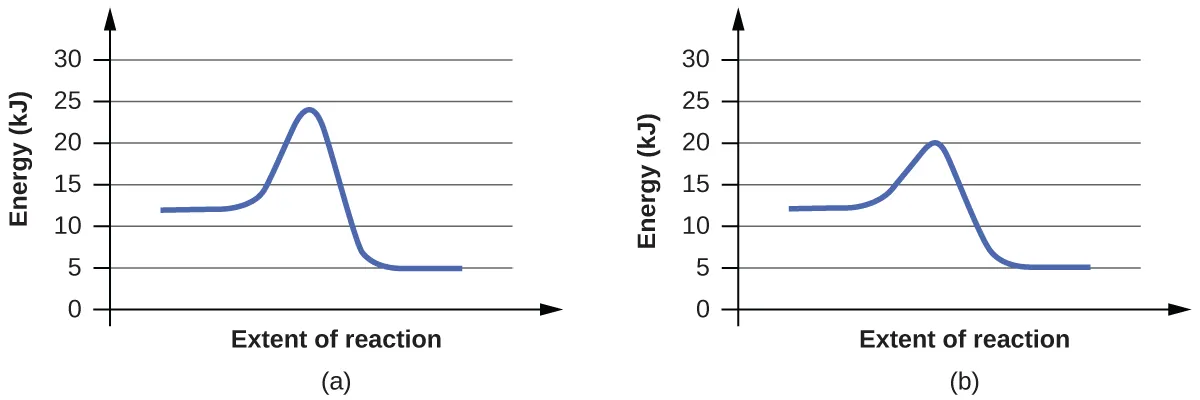(b)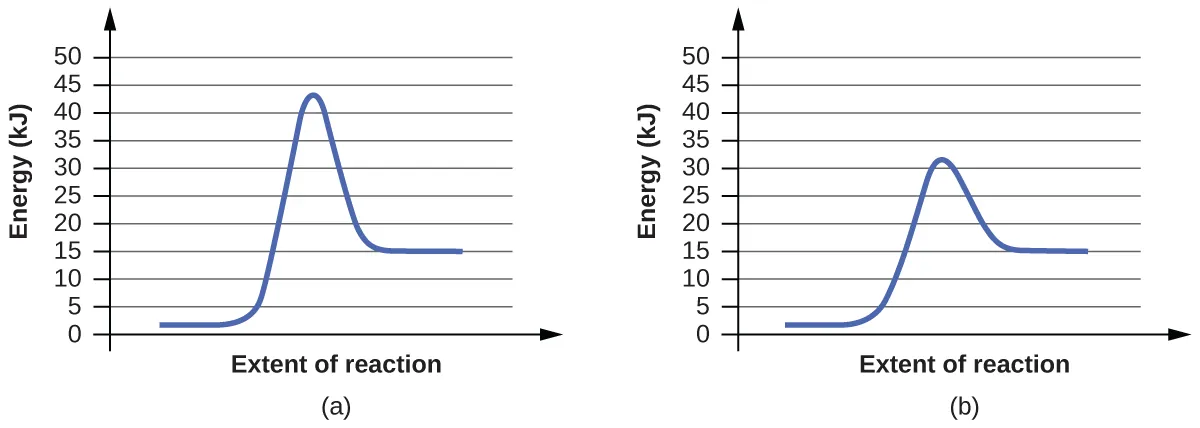80.

For each of the following pairs of reaction diagrams, identify which of the pairs is catalyzed:

(a)(b)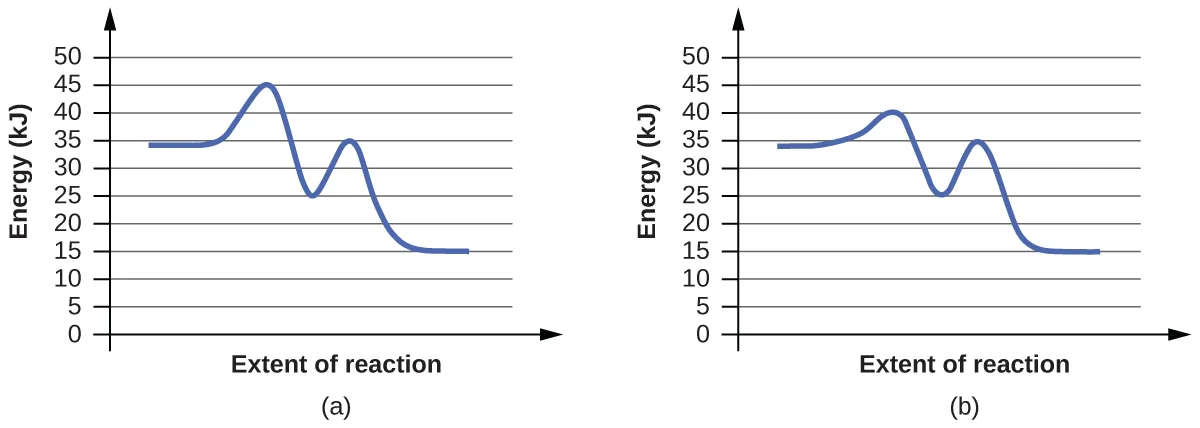81.

For each of the following reaction diagrams, estimate the activation energy (Ea) of the reaction:

(a)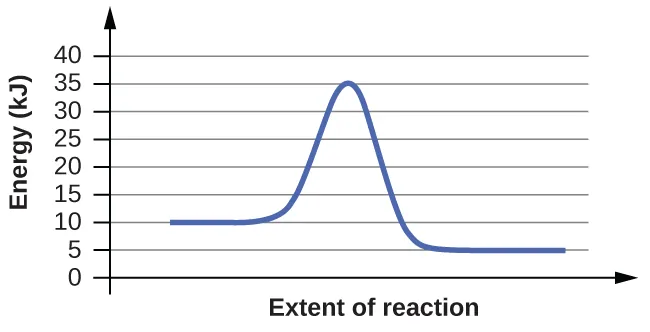(b)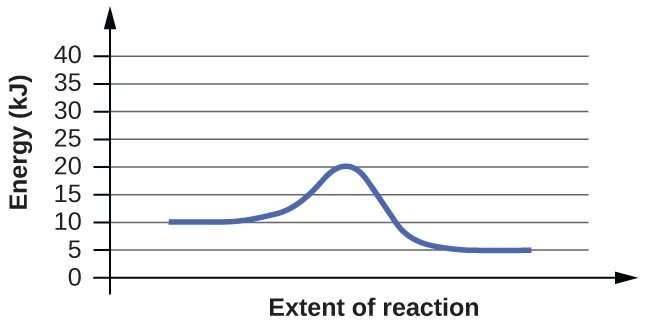82.

For each of the following reaction diagrams, estimate the activation energy (Ea) of the reaction:

(a)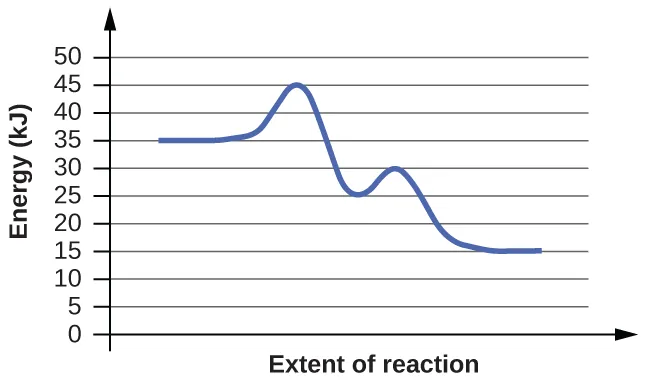(b)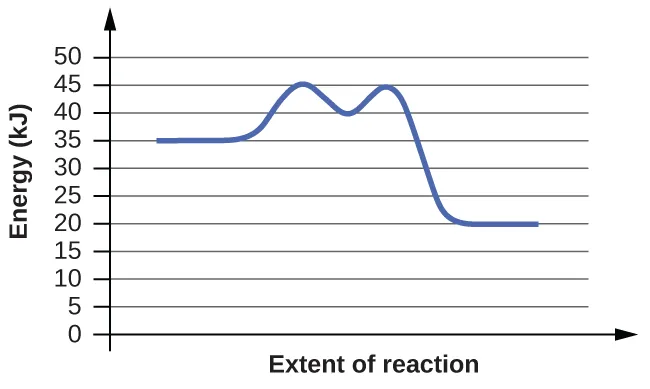83.

Assuming the diagrams in Exercise 12.81 represent different mechanisms for the same reaction, which of the reactions has the faster rate?

84.

Consider the similarities and differences in the two reaction diagrams shown in Exercise 12.82. Do these diagrams represent two different overall reactions, or do they represent the same overall reaction taking place by two different mechanisms? Explain your answer.

### Footnotes

• 2This question is taken from the Chemistry Advanced Placement Examination and is used with the permission of the Educational Testing Service.
Order a print copy

As an Amazon Associate we earn from qualifying purchases.